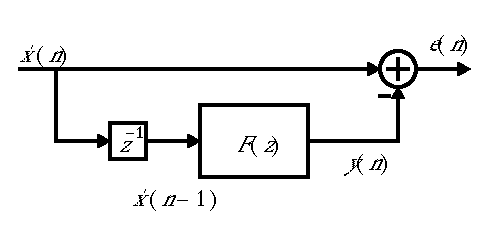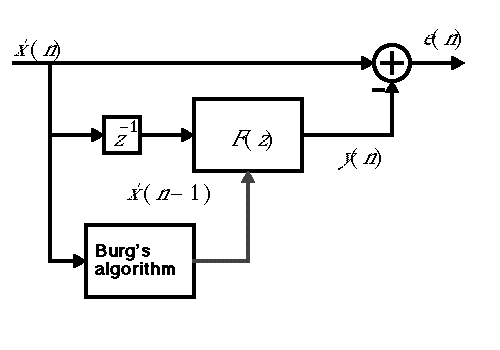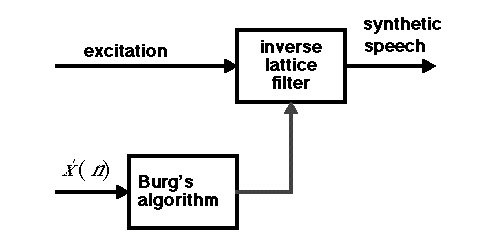EECS20N: Signals and Systems

# Speech Processing Using Linear Prediction

In this set of demonstrations, we illustrate the modern equivalent of the 1939 Dudley vocoder demonstration. Instead of a bank of bandpass filters, modern vocoders use a single filter (usually implemented in a so-called lattice filter structure). The filter coefficients are calculated using any of a number of algorithms (based on linear prediction). In the examples below, we use an algorithm due to John Burg to calculate the filter coefficients.

The original speech signal If you were able to run applets, you would have a button here that would play a sound. , borrowed from the Voder demo, is sampled at 8kHz. The signal is broken into segments of 160 samples (20ms). Each segment is analyzed using Burg's algorithm for its spectral content (a tenth order linear predictor is the result of this analysis).

A linear predictor uses observations of a signal to try to predict the next sample of the signal beyond those it can observe. The overall structure is as shown:The input signal, x'(n), is delayed by one sample by the block labeled z-1. The block labeled F(z) is a filter whose output y(n) is an estimate of the current value of x'(n). Since that block only sees a delayed version of x'(n), its output is a prediction. The error in the prediction, e(n), is the difference between the what is being predicted and the prediction.

Intuitively, linear prediction exploits the fact that a new sample of a signal is not totally independent of previous samples, usually. It captures that dependence. As such, when a predictor is working well, the error signal will have little residual correlation between samples. If the input to the linear predictor is the original voder speech signal If you were able to run applets, you would have a button here that would play a sound. , then the error signal If you were able to run applets, you would have a button here that would play a sound. is not very intelligible. Intelligibility, therefore, must somehow depend on the correlation between samples in the signal.

In fact, for speech, the linear predictor has to constantly change to adapt to what is being said. The input signal is divided into 20ms segments, and each segment is analyzed to provide the coefficients of the prediction filter, as shown below:A box labeled "Burg's algorithm" uses one of several methods for calculating the coefficients of the linear predictor each 20ms.

We can check the hypothesis that intelligibility depends on the correlation between samples by introducing the correlation into some random signal that has no speech content. Suppose we start with a white noise signal If you were able to run applets, you would have a button here that would play a sound. and filter it with the inverse of the prediction error filter above (changing the filter coefficients every 20ms). The result will be intelligible whispered speech If you were able to run applets, you would have a button here that would play a sound. . The block diagram used to create this synthetic speech is as follows:Burg's algorithm is used again to analyze each 20ms segment of speech, but now the results of the analysis are loaded into an "inverse lattice filter," which implements the inverse of the filter above that produced the prediction error.

Notice that since the result is intelligible, information about intelligibility is almost entirely in the results produced by Burg's algorithm. Thus, such an algorithm can (and often does) form the front end of any device that analyzes speech, such as speech recognition system or a speech encoder.

The whispered speech effect above, while intelligible, sounds, well ... whispered. The problem here is that the excitation, white noise, does not match well what the human vocal cords do. The human vocal cords, which provide an excitation signal in natural speech, vibrate at a frequency that depends on the speaker (and whether the speaker is male or female) and on the inflection intended by the speaker. A naive way to try to replicate the effect of the vocal cords is to use a sinusoidal excitation instead of white noise. The result If you were able to run applets, you would have a button here that would play a sound. is completely unintelligible. There is simply not enough spectral richness in a sinusoid.

An alternative excitation that is more spectrally rich is a periodic sequence of impulses, which looks like this:If we set the period at 40 samples (5ms, or 39 zero-valued samples for every non-zero sample), then the excitation If you were able to run applets, you would have a button here that would play a sound. has a perceptual pitch of 200Hz, but obviously no discernable speech content. Filtering it with the inverse lattice filter yields intelligible, if mechanical sounding speech If you were able to run applets, you would have a button here that would play a sound. . Increasing the period to 80 samples results in speech with a lower tone If you were able to run applets, you would have a button here that would play a sound. .

With a periodic pulse excitation, speech sounds very mechanical. A slightly better result If you were able to run applets, you would have a button here that would play a sound. comes from combining white noise with periodic pulses. More sophisticated techniques, such as those used today in digital cellular phones, analyze the speech further to construct much better excitation signals.

Since the intelligibility information is contained in the coefficients produced by Burg's algorithm, we can manipulate the speed of the speech by manipulating these coefficients. For example, if we use every set of coefficients to reconstruct 40ms worth of speech rather than 20ms, the result is slow speech If you were able to run applets, you would have a button here that would play a sound. . Note that we could also get slow speech by using every speech sample twice, but the result If you were able to run applets, you would have a button here that would play a sound. is very different, having the overall pitch shifted down by a factor of two in addition to having the speech slowed down.

We can similarly speed up the speech by using each set of coefficients to reconstruct 10ms worth of speech rather than 20ms, the result is fast speech If you were able to run applets, you would have a button here that would play a sound. . Note that we could also get fast speech by discarding every second speech sample, but the result If you were able to run applets, you would have a button here that would play a sound. is very different, having the overall pitch shifted up by a factor of two in addition to having the speech speeded up.

Finally, synthesizing the speech from a musical excitation If you were able to run applets, you would have a button here that would play a sound. in this case the first few bars of Passio Domini nostri by Arvo Part, yields a particularly interesting result If you were able to run applets, you would have a button here that would play a sound.